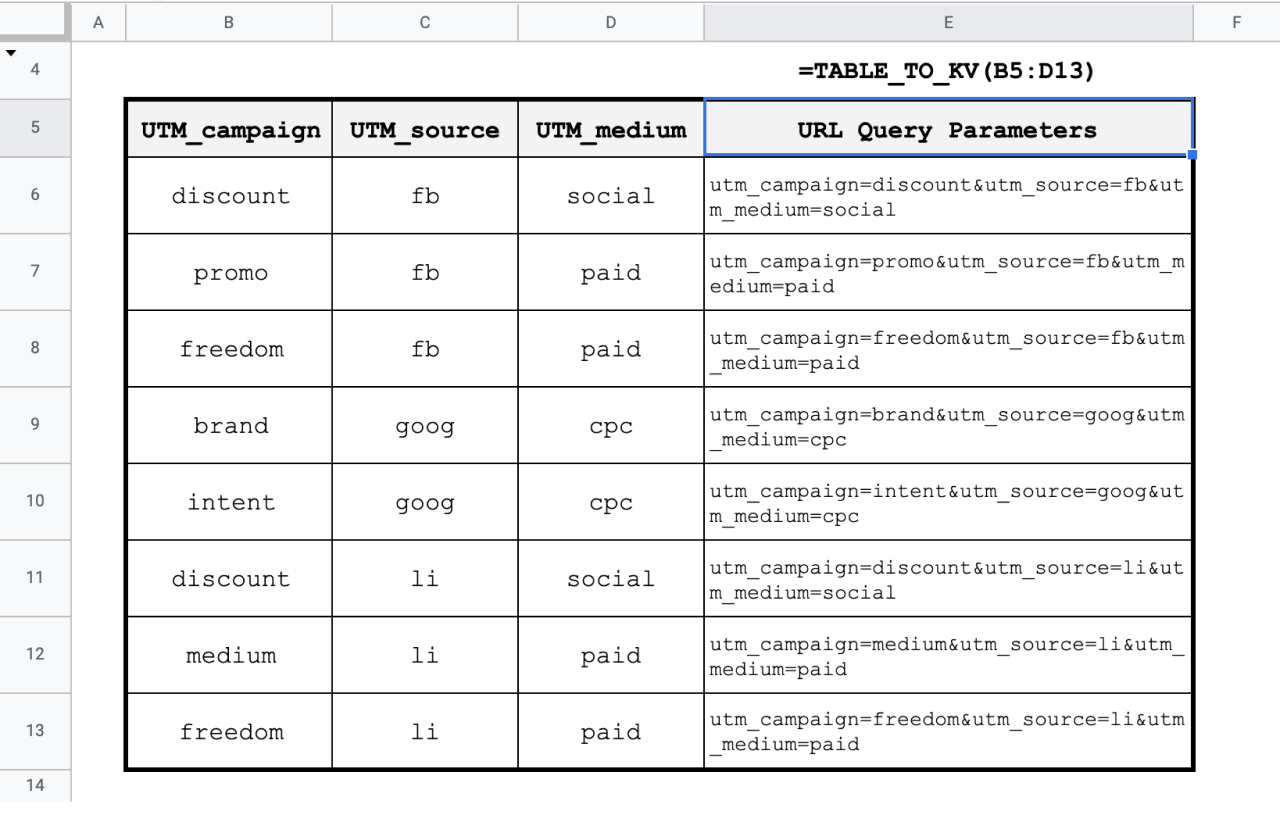# Convert a Google Sheets range into URL key & value parameters with TABLE_TO_KV

Jul 7

The TABLE_TO_KV function is a tool for building URLs our any key & value string quickly and properly.

The most common use case is for building trackable campaign links.

### Function Signature

`=TABLE_TO_KV(table, key_location, no_key_encode, no_value_encode)`

### Argument Definitions

input [range]

• required - range of cells to transform with the keys included in the range.

key_location [number, default = 0]

The location of the key names, or headers.

• 0 - first row
• 1 - last row (bottom row)
• 2 - first column
• 3 - last column (far-right column)

no_key_encode [boolean, default = false]

• Keys are underscore encoded and pushed to lower-case by default. Turn off this feature by setting no_key_encode to true.

no_value_encode [boolean ,default = false]

• By default, URL encodes keys by replacing unsafe ASCII characters with a "%" followed by two hexadecimal digits. When TRUE, the values aren't transformed.

## Use the Cheat

Click the Code Box to Copy

Cheat copied to clipboard ✂️👍
``` /** * Convert table to key-value pairs. * By default, uses URL encoding. * * @param {array} table - key, value table of data to transform * @param {int} key_location - JSON key locations. Default is 0 "first row". * 0 - first row * 1 - last row (bottom row) * 2 - first column * 3 - last column (right) * * @param {boolean} no_encode_keys - false by default, TRUE will turn off underscore transformation on keys * @param {boolean} no_value_encode - false by default, TRUE will turn off encoding on values * * @return Key/Value string for each row in cells * @customfunction */ function TABLE_TO_KV(table, key_location = 0, no_encode_keys = false, no_value_encode = false) { let processed = table_to_object(table, key_location, no_encode_keys); let items = processed.items; let final = []; let return_row = []; for (let i=0; i < items.length; i++) { let obj = items[i]; let params = []; for (let k in obj) { let key = no_encode_keys ? k : encodeURIComponent(k); let value = no_value_encode ? obj[k] : encodeURIComponent(obj[k]); params.push(key + "=" + value); } return_row.push(params.join("&")); if (key_location < 2 || i == (items.length - 1)) { final.push(return_row); return_row = []; } } return final; } function table_to_object(table, key_location = 0, no_encode_keys = false, keep_empty_keys = false, field_indexes) { key_location = Math.min(key_location, 3); let smart_encode_keys = !no_encode_keys; let row_based = true; keep_empty_keys = !!keep_empty_keys || false; // verify we have valid table dimensions if (key_location == 0 || key_location == 1) { // row-based key definition if (!table || table.length < 2 || table.length < 1) { return "Error: Invalid dimensions for 1st Argument 'table'. Table with row-based definitions, must have at least two rows and one column." } } else { row_based = false; if (!table || !table.length || table.length < 2) { // we assume header return "Error: Invalid dimensions for 1st Argument 'table'. Table with column-based definitions, must have at least two columns and one row." } } let keys = []; let row_start = 1; let col_start = 1; let row_end = table.length; let col_end = table.length; switch (key_location) { case 0: keys = table; row_start = 1; row_end = table.length; col_start = 0; col_end = table.length; break; case 1: keys = table[table.length-1]; row_start = 0; row_end = table.length-1; col_start = 0; col_end = table.length; break; case 2: keys = pull_column_(table, 0); row_start = 0; row_end = table.length; col_start = 1; col_end = table.length; break; case 3: keys = pull_column_(table, table.length -1); row_start = 0; row_end = table.length; col_start = 0; col_end = table.length-1; break; default: return "Error: Optional 2nd Argument Invalid for 'key_location'. Expecting value 0 - 3"; } // clear empty keys keys = keys.filter(function(el) { return !!el; }); if (typeof(field_indexes) == "string" ) { field_indexes = [[field_indexes]]; } if (smart_encode_keys) { // re-encode keys and field_index references keys.forEach(function(v, index) { this[index] = smart_url_encode_(v); }, keys); if (field_indexes) { field_indexes.forEach(function(v, index) { this[index] = smart_url_encode_(v); }, field_indexes); } } let json = []; let out_start, out_end, inner_start, inner_end; out_start = row_based ? row_start : col_start; out_end = row_based ? row_end : col_end; inner_start = row_based ? col_start : row_start; inner_end = row_based ? col_end : row_end; for (let i=out_start; i < out_end; i++) { let o = {}; let ki = 0; let empty = true; for (let j=inner_start; j < inner_end; j++) { let t = row_based ? table[i][j] : table[j][i]; if (t != "") { empty = false; } else if (!keep_empty_keys) { ki++; continue; } else if (t !== 0 && t !== false) { t = null; } // convert date to time in millis if (t instanceof Date) { t = t.getTime().toFixed(); } o[keys[ki]] = t; ki++; } if (!empty) { json.push(o); } } let indexes = {}; if (field_indexes) { for (let i=0; i < field_indexes.length; i++) { let is_last_element = i == field_indexes.length-1; let index = field_indexes[i]; if (!keys.includes(index)) { Logger.log(`\${index} not found in keys`); continue; } let io = {}; for (let r=0; r < json.length; r++) { let item = json[r]; let val = item[index]; if (val) { if (!io[val]) { io[val] = []; } io[val].push(r); } } indexes[index] = io; } } let ret = { "items": json, "fields": keys, "meta": { count: json.length } }; if (indexes){ ret["field_indexes"] = indexes; } return ret; } /** * URL Encodes * * @param {string} input - input to transform * @param {boolean} keep_case - default false, if true it doesn't lower case * * @return a smart encoded string for URLs that makes them safe * */ function smart_url_encode_(input, keep_case = false) { if (!keep_case) { input = input.toLowerCase(); } return input .trim() .replace(/\s+/ig, "_") .replace(/\W/ig, "") } function pull_column_(table, col){ const column = []; for(let i=0; i < table.length; i++) { column.push(table[i][col]); } return column; } /** * Generates a guaranteed unique ID * * @customfunction */ function ID() { return Utilities.getUuid(); }```Cyrus Radfar